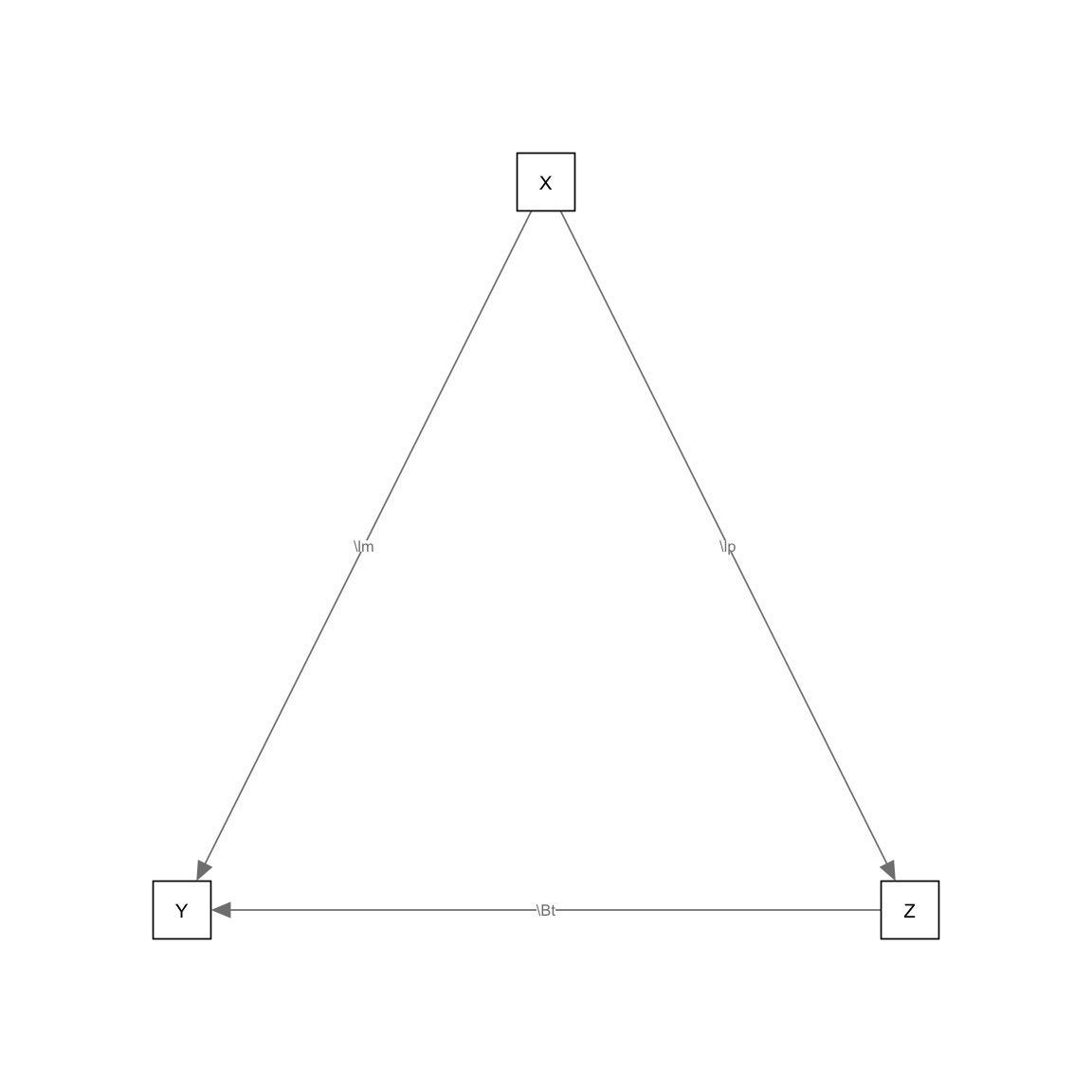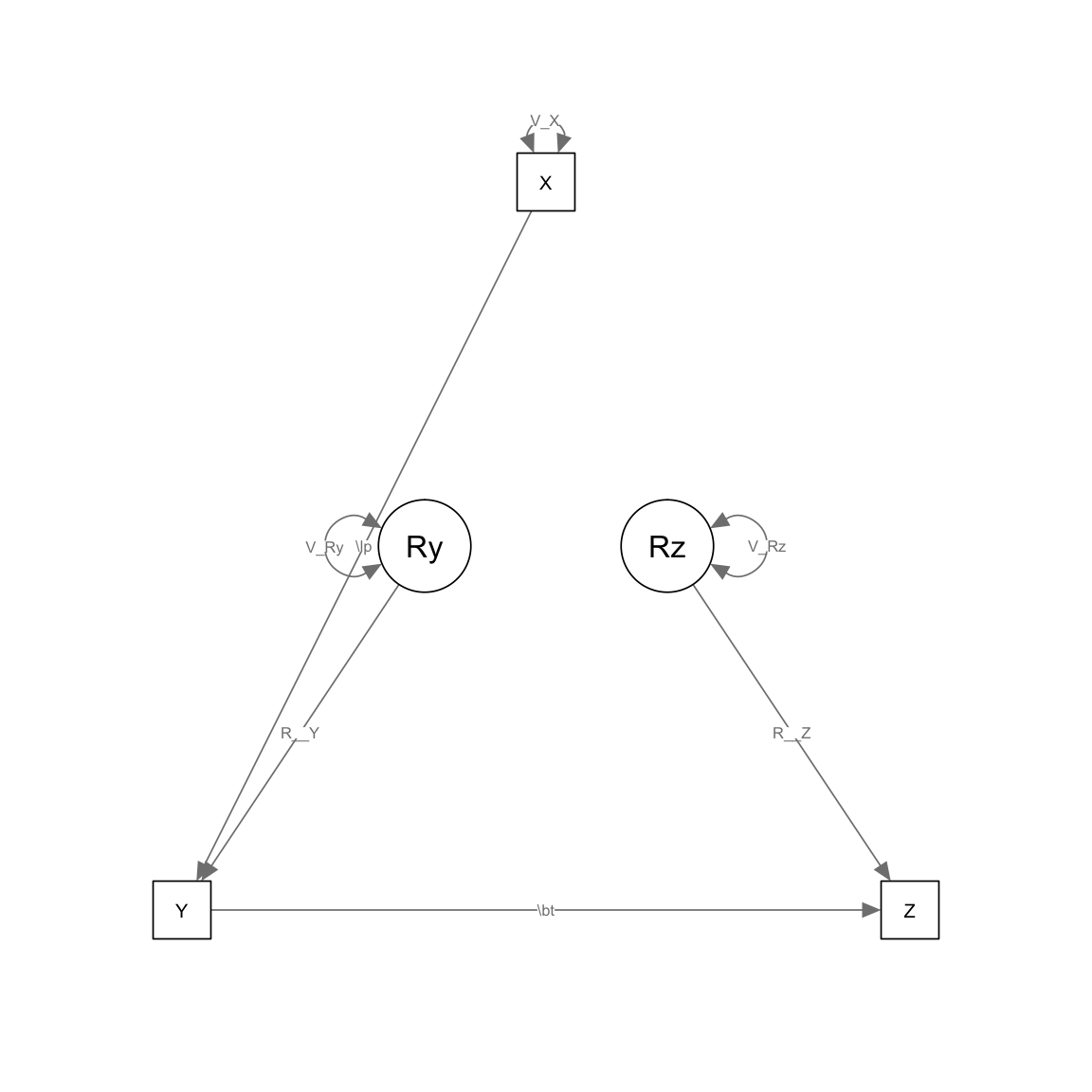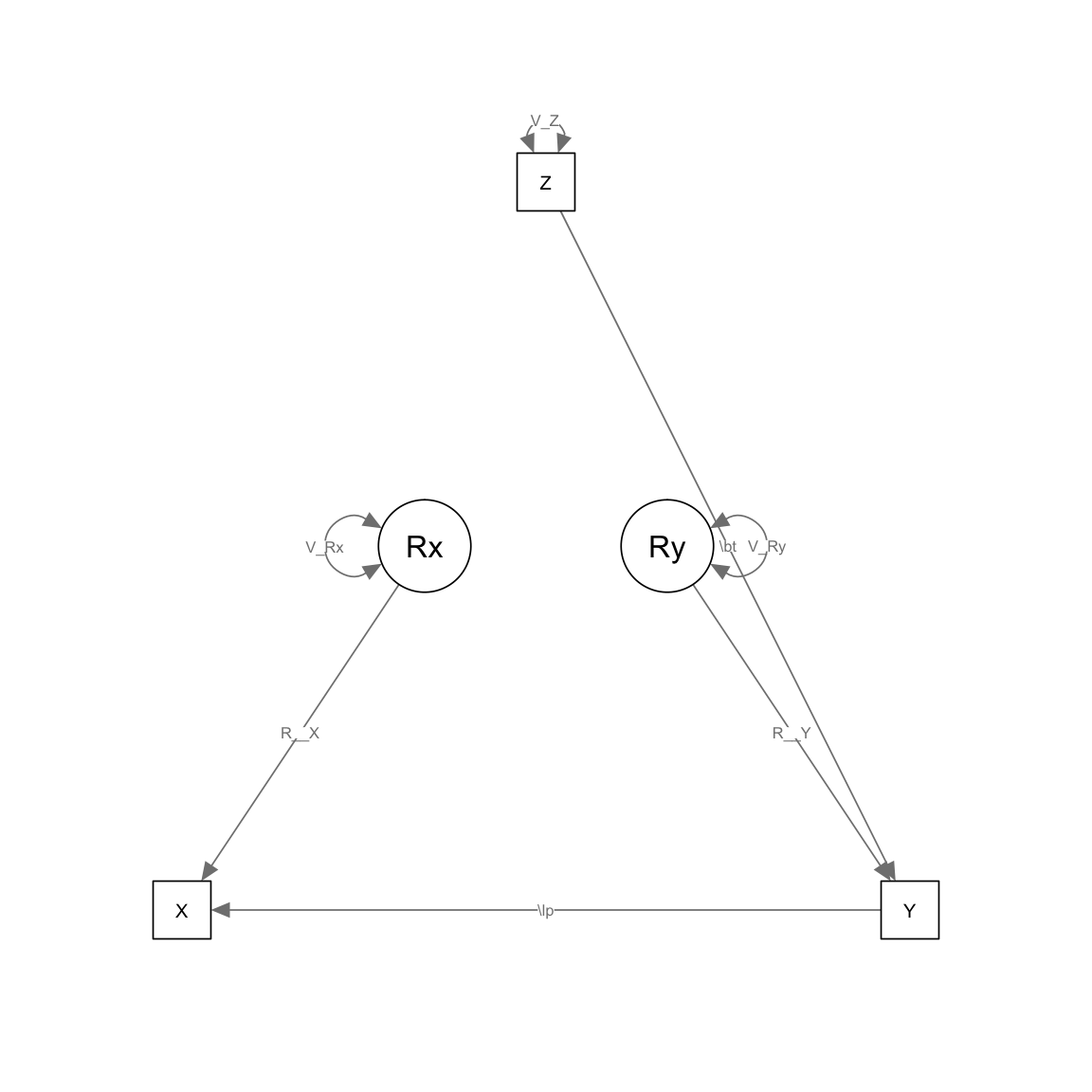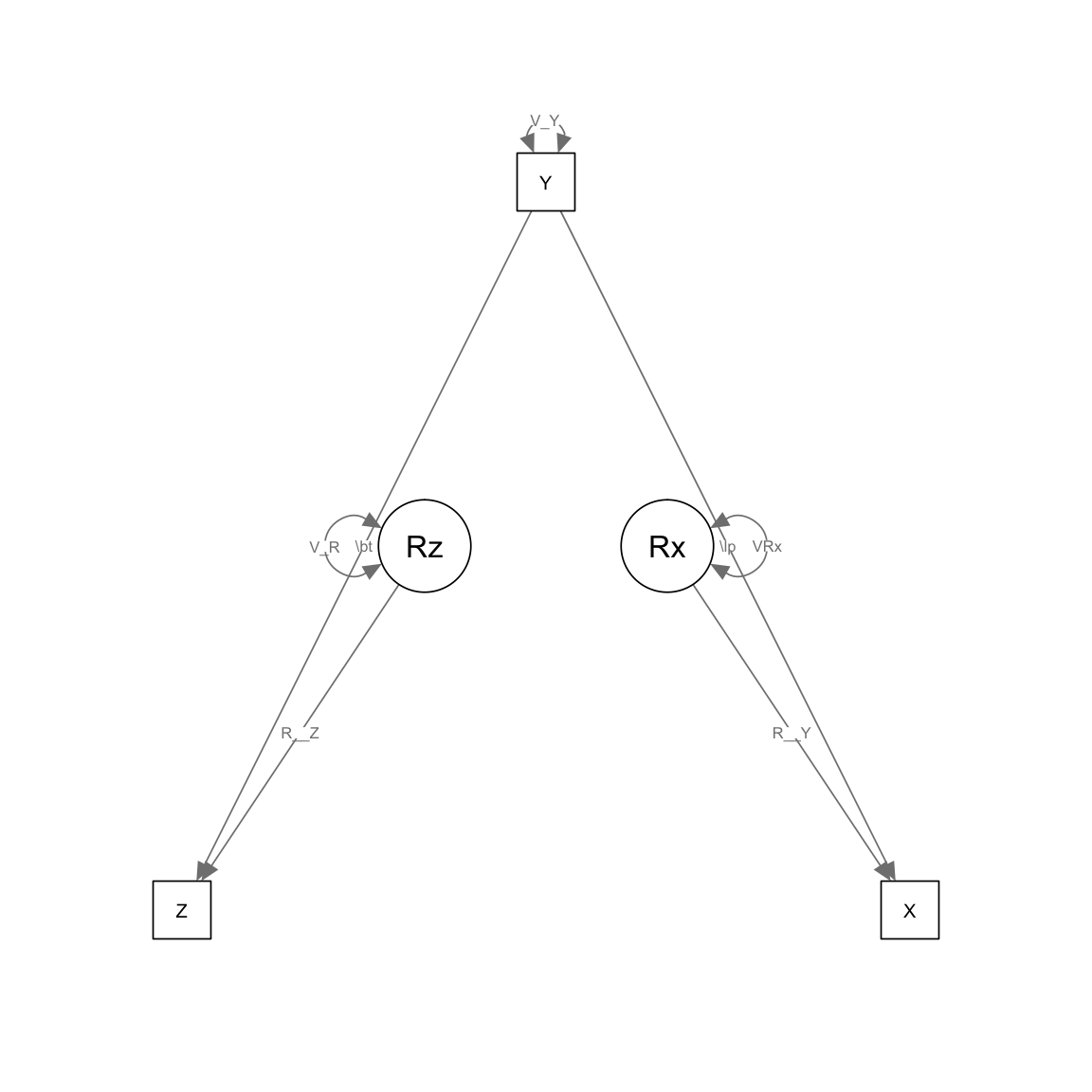This document carries the code and output used in Brick and Bailey (submitted). Specifically, we illustrate the use of Matrices of Implied Causation using a mediation model and three examples from the literature. They are:

1. A dummy mediation model
2. Three models from Tomarken & Waller (2003), in this case simplex model variants in their Example 1.
3. CLPM in comparison to RI-CLPM from Bailey et al., 2007.
4. RI-CLPM intervention

# Setup and packages

First we need MICr, OpenMx, and also some tools for plotting.

library(MICr)
library(OpenMx)
# Turn on plots if semPlot is available:
if(library(semPlot, logical.return=TRUE))  drawPlots<-TRUE else drawPlots <- FALSE 

# Example 0: Pedagogical mediation model

The first is a dummy example:

XYZmodel <- mxModel("XYZ", manifestVars=c("X", "Y", "Z"), type="RAM",
mxPath(from="Z", to="Y", values=.6, labels="\\Beta"),
mxPath(from="X", to=c("Y", "Z"), values=c(.2, .8), labels=c("\\lambda", "\\alpha")),
mxMatrix("Iden",3,name="I"))

Whenever we run a model like this, I’ll add a sempaths plot. These models are complex, so the plot generated there is rarely a good view of what the model should look like. The figures in the paper itself are a better view of the models, but for generation purposes we create them here. They are generated with Onyx (see http://onyx.brandmaier.de/ for more), although some are modified for readability.

if(drawPlots) semPaths(XYZmodel)We can render the MIC for this model directly.

MIC(XYZmodel)
     X Y   Z
X 1.00 0 0.0
Y 0.68 1 0.6
Z 0.80 0 1.0

The MIC is read like the A matrix in a RAM model: the path from X to Z is in the column for X and the row for Z (here, it is .8). As expected, this shows the total effect–the model-implied causal influence–from each variable to each other variable.

This can be rendered more succinctly as a MIC Table:

MICTable(XYZmodel)


Table: Implied Causation Table: XYZ

from   to     XYZ
-----  ---  -----
X      Z     0.80
X      Y     0.68
Z      Y     0.60

# Example 1: Three models from Tomarken & Waller (2003)

The first example shows three cases from Tomarken & Waller’s 2003 paper. These three models fit identically, but make very different causal predictions.

## Tomarken Example 1A:

The first model is a

tomarken1A <- mxModel("Tomarken1A",
type="RAM", manifestVars = c("X", "Y", "Z"), latentVars = c("Ry", "Rz"),

# Latent to manifest paths
mxPath(from="Ry", to="Y",  free=FALSE, value=1.0, label=c("Ry_to_Y") ),
mxPath(from="Rz", to="Z",  free=FALSE, value=1.0, label=c("Rz_to_Z") ),

# Manifest chain
mxPath(from="X",  to="Y",  free=TRUE, values=0.8, labels=c("\\alpha") ),
mxPath(from="Y",  to="Z",  free=TRUE, values=0.8, labels=c("\\beta") ),

# Variances
mxPath(from=c("Ry", "Rz", "X"),
arrows=2, free=TRUE, values=1.0,
labels=c("V_Ry", "V_Rz", "V_X") )
)

Sempaths plots of these models show the differences fairly well.

if(drawPlots) semPaths(tomarken1A)## Tomarken Example 1B

Model 1B resembles model 1A, but the chain flows in the opposite direction, with Z predicting Y and Y predicting X.

tomarken1B <- mxModel("Tomarken1B",
type="RAM", manifestVars = c("X","Y","Z"), latentVars = c("Rx", "Ry"),

# Latent to manifest paths
mxPath(from="Ry", to="Y",  free=FALSE, value=1.0, label=c("Ry_to_Y") ),
mxPath(from="Rx", to="X",  free=FALSE, value=1.0, label=c("Rx_to_X") ),

# Manifest chain
mxPath(from="Z",  to="Y",  free=TRUE, values=0.8, labels=c("\\beta") ),
mxPath(from="Y",  to="X",  free=TRUE, values=0.8, labels=c("\\alpha") ),

# Variances
mxPath(from=c("Ry", "Rx", "Z"),
arrows=2, free=TRUE, values=1.0,
labels=c("V_Ry", "V_Rx", "V_Z") )
)
# Throws a warning because the model has not been run.
if(drawPlots) semPaths(tomarken1B)## Tomarken Example 1C

The last Tomarken example model is a fork, with Y predicting X and Z.

tomarken1C <- mxModel("Tomarken1C",
type="RAM", manifestVars = c("X","Y","Z"), latentVars = c("Rz","Rx"),

mxPath(from="Y", to=c("Z","X"), free=TRUE, values=.8, labels=c("\\beta","\\alpha") ),

# Latent residual terms
mxPath(from="Rz", to="Z",  free=TRUE, value=1.0, label="Rz_to_Z" ),
mxPath(from="Rx", to="X",  free=TRUE, value=1.0, label="Ry_to_Y" ),

mxPath(from=c("Y", "Rx", "Rz"),
arrows=2, free=FALSE, values=1.0,
labels=c("V_Y", "VRx", "V_Rz"))
)
# Throws a warning because the model has not been run.
if(drawPlots) semPaths(tomarken1C)## Tomarken MICs

The MICs can be rendered directly as matrices.

MIC(tomarken1A)
      X   Y Z  Ry Rz
X  1.00 0.0 0 0.0  0
Y  0.80 1.0 0 1.0  0
Z  0.64 0.8 1 0.8  1
Ry 0.00 0.0 0 1.0  0
Rz 0.00 0.0 0 0.0  1
MIC(tomarken1B)
   X   Y    Z Rx  Ry
X  1 0.8 0.64  1 0.8
Y  0 1.0 0.80  0 1.0
Z  0 0.0 1.00  0 0.0
Rx 0 0.0 0.00  1 0.0
Ry 0 0.0 0.00  0 1.0
MIC(tomarken1C)
   X   Y Z Rz Rx
X  1 0.8 0  0  1
Y  0 1.0 0  0  0
Z  0 0.8 1  1  0
Rz 0 0.0 0  1  0
Rx 0 0.0 0  0  1

A more succinct approach is to plot the MICTable that compares all three. Because the residuals are not of particular interest, we focus only on the influences from and to X, Y, and Z. Note that this table differs in its sorting function from the one displayed in the paper.

MICTable(tomarken1A, tomarken1B, tomarken1C, from=c("X", "Y", "Z"), to=c("X", "Y", "Z"))


Table: Implied Causation Table: Tomarken1A, Tomarken1B, Tomarken1C Existence & Sign & Scale

from   to    Tomarken1A   Tomarken1B   Tomarken1C
-----  ---  -----------  -----------  -----------
X      Y           0.80         0.00          0.0
Y      Z           0.80         0.00          0.8
X      Z           0.64         0.00          0.0
Y      X           0.00         0.80          0.8
Z      X           0.00         0.64          0.0
Z      Y           0.00         0.80          0.0

# Example 2: CLPMs from Duncan, et al.

Example 2 comes from Duncan(2007) courtesy of Bailey et al. (2018?). Three fairly intricate models, and we’ll do one better by dropping the person-level intercepts.

First, need the data and the correlation matrix–it’s in the paper, luckily.

means <- c(68.08, 93.09, 107.40, 116.80, 49.91, 73.87, 90.65, 102.87)
times <- c("K", 1:3)
nTimes <- length(times)

manifests <- paste(rep(c("Read", "Math"), each=nTimes), rep(times, 2), sep="")
occasions <- paste(rep(c("OR", "OM"), each=nTimes), rep(times, 2), sep="")
sds <- c(14.17, 18.02, 15.51, 14.82, 12.84, 17.64, 16.66, 15.63)
corMat <- matrix(c(
1.00, 0.79, 0.71, 0.64, 0.73, 0.66, 0.62, 0.59,
0.79, 1.00, 0.86, 0.78, 0.71, 0.73, 0.70, 0.67,
0.71, 0.86, 1.00, 0.85, 0.69, 0.71, 0.74, 0.69,
0.64, 0.78, 0.85, 1.00, 0.67, 0.70, 0.73, 0.72,
0.73, 0.71, 0.69, 0.67, 1.00, 0.83, 0.78, 0.75,
0.66, 0.73, 0.71, 0.70, 0.83, 1.00, 0.86, 0.82,
0.62, 0.70, 0.74, 0.73, 0.78, 0.86, 1.00, 0.88,
0.59, 0.67, 0.69, 0.72, 0.75, 0.82, 0.88, 1.00), nrow=8)
covMat <- diag(sds) %&% corMat
names(means) <- manifests
dimnames(covMat) <- list(manifests, manifests)
dimnames(corMat) <- list(manifests, manifests)

## Duncan CLPM1

ModelCLPM1 <- mxModel("CLPM1", type="RAM", manifestVars = manifests,

mxPath(from=occasions, to=manifests,
arrows=1, values=1, free=FALSE),

# Occasion variances
mxPath(from=occasions, arrows=2, values=1, free=TRUE,
labels=paste0("V_", occasions), lbound=.1),

# Covariation of error/process
mxPath(from=occasions[1:4], to=occasions[5:8],
arrows=2, free=TRUE, values=.25,
labels=paste0("ORM_", times)),

mxPath(from=occasions[1:3], to=occasions[2:4],
arrows=1, free=TRUE, values=.5,
labels=paste0("AR_R_", times[1:3])),

# AR of Math
mxPath(from=occasions[5:7], to=occasions[6:8],
arrows=1, free=TRUE, values=.3,
labels=paste0("AR_M_", times[1:3])),

# Cross-lagged R->M
mxPath(from=occasions[1:3], to=occasions[6:8],
arrows=1, free=TRUE, values=.10,
labels=paste0("CL_R", times[1:3], "_M", times[2:4])),

# Cross-lagged M->R
mxPath(from=occasions[5:7], to=occasions[2:4],
arrows=1, free=TRUE, values=.03,
labels=paste0("CL_M", times[1:3], "_R", times[2:4])),

mxData(type="cor", observed = corMat, numObs = 9612)
)
CLPM1 <- mxTryHard(ModelCLPM1)
if(drawPlots) semPaths(CLPM1)